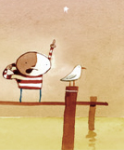###Author Topic: Turn off texture blending / strange lines in model texture  (Read 1437 times)

####FutureCow

• HelpEditor
• Prof. Inline
••• Posts: 680##### Turn off texture blending / strange lines in model texture
« on: 2012-Jun-10 »
I'm trying a new approach to texturing a model (a generated 3D landscape) but I'm having some unexpected behaviour I'm hoping someone will be able to pinpoint for me.

Firstly my model is built as follows
• I load a list of heights of each square tile - the map is 128x128 tiles in size
• I create the model in triangle strips
• I load a test image to use for a texture that is 128x128 pixels in size
• The texture is set to the image that was loaded then the model is drawn

Here's what my model comes out looking like - this is a picture taken from looking straight down at the model. The lines aren't 100% straight as the section of the model shown isn't flat so I'm not worried about that.Here's the texture. Each quad in my model correllates to exactly 1 pixel in the source texture.Here's how I create the model
Code: (glbasic) [Select]
` FOR Z=0 TO (ModelSizeZ-2) ZTexValue1=1/(128/(Z+1))                      // Set the first Z offset into the texture image ZTexValue2=1/(128/(Z+2))                      // Set the second Z offset into the texture image X_OBJNEWGROUP FOR X=0 TO (MapSizeX-2) STEP 2 XTexValue1=1/(128/(X+1))                      // Set the first X offset into the texture image XTexValue2=1/(128/(X+2))                      // Set the second X offset into the texture image       X_OBJADDVERTEX   X,   HeightsArray[X][Z+1],  Z+1,  0,0, RGB(255,255,255)         // Create quad. Bottom left corner         X_OBJADDVERTEX   X,   HeightsArray[X][Z],      Z,  0,1, RGB(255,255,255)              // Top left corner         X_OBJADDVERTEX   X+1, HeightsArray[X+1][Z+1], Z+1, 1,0, RGB(255,255,255)     // Bottom right corner         X_OBJADDVERTEX   X+1, HeightsArray[X+1][Z],    Z,  1,1, RGB(255,255,255)         // Top right Corner ENDIF NEXT NEXT`
My issues
1) Any idea why am I getting the image coming out looking like it's made up of joined up strips? I would've expected everything to blend together nicely, but instead I get the lines in it where two strips of triangles meet.

2) Each tile (ie square of 2 triangles) should be a solid colour. How do I stop each colour from blending into the next one so that each square's doesn't blend into those of the squares next to it? Is this because of the small texture image size? (128x128 pixels)

####erico

• Community Developer
• Prof. Inline
••• Posts: 4396##### Re: Turn off texture blending / strange lines in model texture
« Reply #1 on: 2012-Jun-10 »
I didn´t go through the code yet to understand, but quick shots would be:
1- UVmap problems.

####FutureCow

• HelpEditor
• Prof. Inline
••• Posts: 680##### Re: Turn off texture blending / strange lines in model texture
« Reply #2 on: 2012-Jun-11 »
Hi Erico,
1) This problem just fixed itself, but I'm not sure how. I'll repost if I can figure it out. Thanks anyway!!!
2) SMOOTHSHADING fixed that issue thanks! I didn't spot the command as it's not listed in the 3D commands help section. It should've occurred to me that the command would be relevant to what I'm doing as textures are 2D.

Cheers!